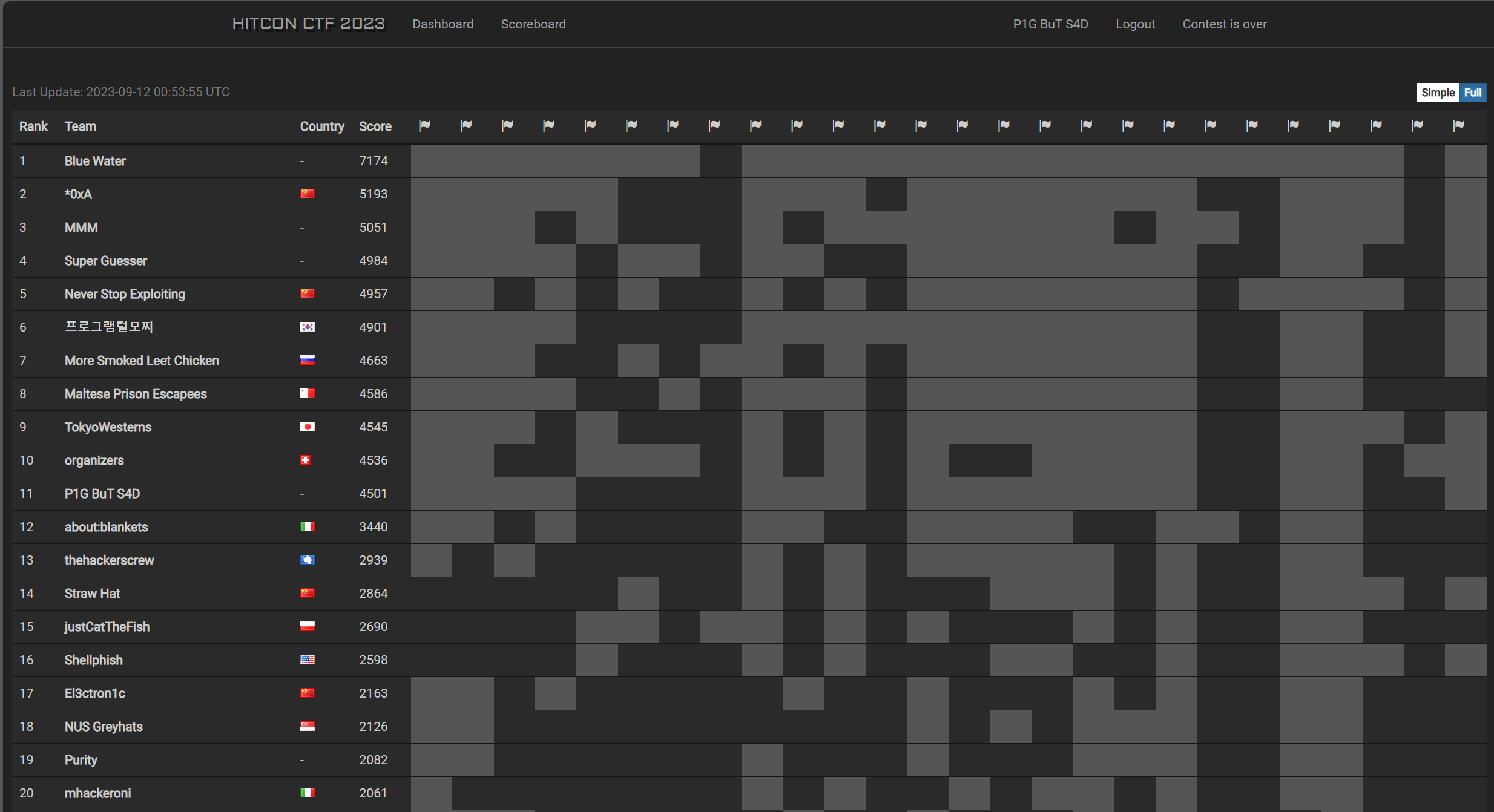September 11, 2023

## WriteUps of HITCONCTF2023(part crypto)HITCONCTF2023 Scoreboard

During this competition, we can learn a lot from maple3142!

## echo(284 pts, 20 solve)

### challenge description

A secure, cryptographically signed echo-as-a-service.

You can use choice 1 to sign a msg with the form: echo {quote(msg)}.

And choice 2 can execute the cmd which you should get its signature.

sign is implemented by RSA(512).

### my solution

At first, we don’t know about the pub parameters of rsa.

I want to compute the modulus n by GCD, like

But there is a problem with this. When you quote a msg with utf-8,there will be ' at the front and back.

the code:

We can get the n by this way.

After that , I want to get e or d but it’s too difficult. I want to do something in the cmd.

./give me flag please can get flag, so I try to construct

cmd = (./give me flag please&' + anything + ') ; sign(cmd) = kn;

anything  should be all bytes in [0,128], LLL can do this.

## ezrsa(327 pts, 11 solve)

### challenge description

I am out of ideas, so why not just make a challenge from a random paper.

a implementation of this paper, but there is a problem in compute_u,which is different with paper’s.

### my solution

1. gcd to get n
2. y^2=x^3%n ⇒ dlp to get 4th condition’s d
3. continued fraction d/(n+1) to get e,cuz e<N^0.125
4. use d and e , the 4th condition a's curve can let u compute the GCD(phi_factor*C,n)==p with a probability 1/16.
5. compute two square of p and q to get priv
6. solve the last prob

## Share(222 pts, 47 solve)

### challenge description

I hope I actually implemented Shamir Secret Sharing correctly this year. I am pretty sure you won’t be able to guess my secret even when I give you all but one share.

### my solution

getRandomRange(0, self.p - 1) the max of this function is p-2, so we can choose small prime p and n=p-1, then we brute the only one value of secret%p which make the other coefficients ∈ [0,p-2].

then crt the value with prod(p_i) > 256 bit. but you should one time send a lot of p and n, cuz the time of interaction.

like this

## Random Shuffling Algorithm(321 pts, 12 solve)

### challenge description

I think you already know what is this challenge about after seeing the challenge name :)

m0,m1,m2 random choose, m3 = flag^m0^m1^m2, msg = [m0,m1,m2]

get 100 pubs (pub(1024 bit) = p*q).

give pubs and cts.

### my solution

then you can crt the to get a polynomial

we know , by coppersmith’s bound, we can compute

beta = 1.0,delta = 44, X = 2**1016; =>1017 = (1024n)(1/44-epsilon), n <= 100;

we choose n=100,epsilon = 0.0125, small root the by flatter, about 15 mins to get the 4 small roots.